# NCERT Solutions For Class 10 Maths Chapter 4 Quadratic Equations Ex 4.2

Get Free NCERT Solutions for Class 10 Maths Chapter 4 Ex 4.2 Quadratic Equations Class 10 Maths NCERT Solutions are extremely helpful while doing homework. Exercise 4.2 Class 10 Maths NCERT Solutions were prepared by Experienced ncert-books.inTeachers. Detailed answers of all the questions in Chapter 4 Maths Class 10 Quadratic Equations Exercise 4.2 Provided in NCERT Textbook

Topics and Sub Topics in Class 10 Maths Chapter 4 Quadratic Equations:

 Section Name Topic Name 4 Quadratic Equations 4.1 Introduction 4.2 Quadratic Equations 4.3 Solution of a Quadratic Equation by Factorisation 4.4 Solution of a Quadratic Equation by Completing the Square 4.5 Nature of Roots 4.6 Summary

You can also download the free PDF of Chapter 4 Ex 4.2 Quadratic Equations NCERT Solutions or save the solution images and take the print out to keep it handy for your exam preparation.

 Board CBSE Textbook NCERT Class Class 10 Subject Maths Chapter Chapter 4 Chapter Name Quadratic Equations Exercise Ex 4.2 Number of Questions Solved 6 Category NCERT Solutions

## NCERT Solutions For Class 10 Maths Chapter 4 Quadratic Equations Ex 4.2

NCERT Solutions for Class 10 Maths Chapter 4 Quadratic Equations Ex 4.2 are part of NCERT Solutions for Class 10 Maths. Here we have given NCERT Solutions for Class 10 Maths Chapter 4 Quadratic Equations Ex 4.2.

Question 1.
Find the roots of the following quadratic equations by factorisation:
(i) x2 -3x – 10 = 0
(ii) 2x2 + x – 6 = 0
(iii) √2x2 + 7x + 5√2 = 0
(iv) 2x2 – x +$\frac { 1 }{ 8 }$= 0 8
(v) 100 x2 – 20 X + 1 = 0
Solution: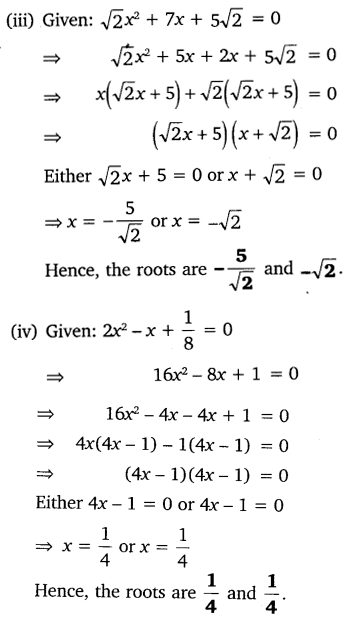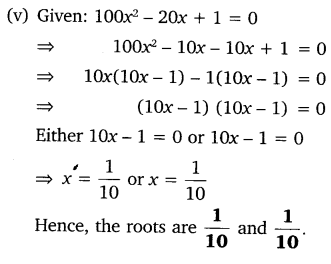Question 2.
Solve the following situations mathematically:
(i) John and Jivanti together have 45 marbles. Both of them lost 5 marbles each and the product of the number of marbles they now have is 124. We would like to find out how many marbles they had to start with.
(ii) A cottage industry produces a certain number of toys in a day. The cost of production of each toy (in rupees) was found to be 55 minus the number of toys produced in a day. On a particular day, the total cost of production was ₹750. We would like to find out the number of toys produced on that day.
Solution: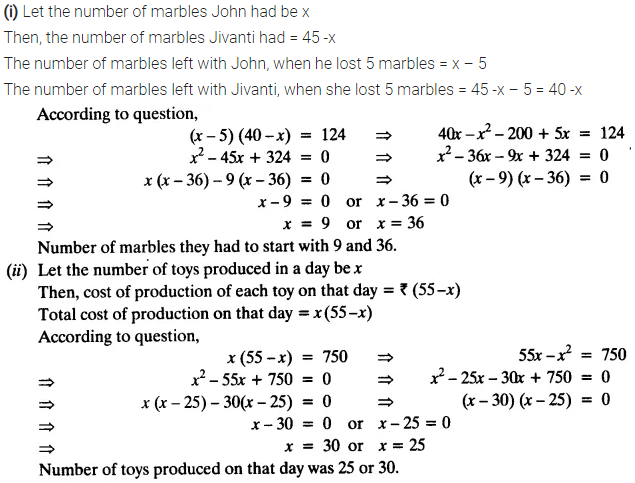Question 3.
Find two numbers whose sum is 27 and product is 182.
Solution:Question 4.
Find two consecutive positive integers, the sum of whose squares is 365.
Solution: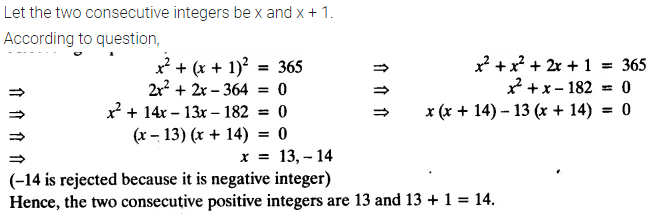Question 5.
The altitude of a right triangle is 7 cm less than its base. If the hypotenuse is 13 cm, find the other two sides.
Solution:Question 6.
A cottage industry produces a certain number of pottery articles in a day. It was observed on a particular day that the cost of production of each article (in rupees) was 3 more than twice the number of articles produced on that day. If the total cost of production on that day was ₹90, find the number of articles produced and the cost of each article.
Solution: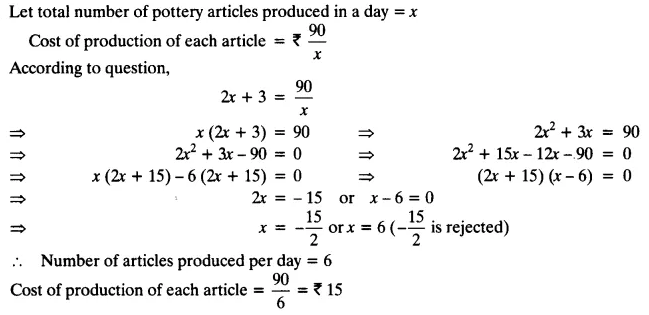error: Content is protected !!
+